## What is ISBLANK?

The Excel ISBLANK function is to check whether a cell is blank and returns TRUE if it is or FALSE if it is not.

To deal with the financial data during the process of performing financial forecasting, reporting, and operational metrics tracking; analyzing financial data, and creating financial models, the ISBLANK function is one of the most important functions in financial analysis, it is very useful for checking whether a specific cell is blank or not. It helps remove both regular and non-breaking space characters.

Syntax:

=ISBLANK (value)

## Key Learning Points

• Purpose: the ISBLANK function is to test if a cell is empty.
• Parameters: the function only requires one argument- the value to check. It could be blank (empty cell), error value(#VALUE!), logical value, text, number, quoted value, or name.
• Return value: the ISBLANK function will return a logical value, if the cell is blank, the return value will be TRUE; or else, it will be FALSE.

## More Detail

Empty string: the real meaning of the ISBLANK function is “is empty”. For example, if a cell contains space or any formula which makes the cell look blank but in fact not empty, as a result, ISBLANK will return FALSE instead of TRUE. This can cause problems when the goal is to count or process blank cells that include empty strings.

One workaround for this situation is to use the LEN function, which is used to test for a length of zero.

=LEN(A28)=0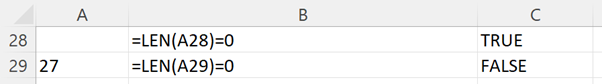The return result will be TRUE if A28 is empty or contains a formula that returns an empty string. In this way, the same effect can be achieved as the ISBLANK function.

## Example

Below is a list of cells that contains a different value. If the cell contains nothing at all, the ISBLANK function will return TRUE; if it contains any value, including any space, text, number, date, time, month, year, percentage, logical value, formula or even error value, ISBLANK will return FALSE.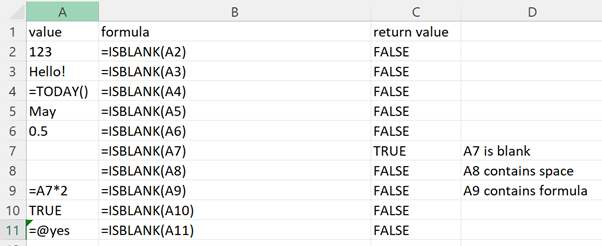Cell A2 contains the number value 123, and ISBLANK returns the result as FALSE, which means CELLA2 is not blank. Taking Cell A8 as an example, although it looks blank, Cell A8 contains space so ISBLANK returns the result as FALSE; Cell A7 is a real blank cell so the return result is TRUE.

Sometimes you may not want a formula to display a TRUE or FALSE result, instead, you may just want to check if the cell is blank, in which case, you can use IF nested with the ISBLANK function:

=IF(ISBLANK(A15),”Blank”,”Not Blank”)

This means if A15 is blank, then return “Blank”, otherwise, return “Not Blank”.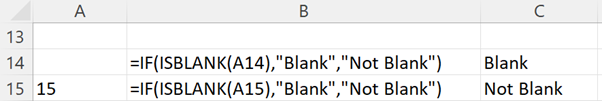The ISBLANK can be replaced with an empty string( “”), which basically means “nothing”, with Excel’s math operations “=” or “<>”. And these two can be used interchangeably, which means ISBLANK(A1) is equivalent to A1=””. Likewise, NOT(ISBLANK(A1)) is the same as A1<>, however, the return result is the opposite to the =ISBLANK(A1).

=A1=””

Which will return TRUE if A1 is empty

=A1<>””

Which will return TRUE if A1 is not empty

The formula below means if the CellA16 contains nothing, then return “Blank”, otherwise “Not Blank”).

=IF(A16=””,”Blank”,”Not Blank”)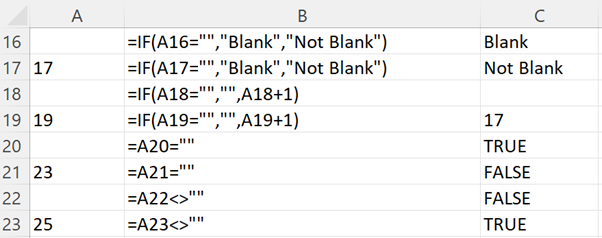In the above table Cell A16 is empty, the formula  =IF(A16=””,”Blank”,”Not Blank”) returns the results as “Blank”.  Cell A17 contains the number 17, which means it is not empty, as a result, the =IF(A17=””,”Blank”,”Not Blank”) returns the results as “Not Blank”.

ISBLANK can also be nested inside the NOT function and it will return TRUE when a cell is not empty, and FALSE when a cell is empty.

=NOT(ISBLANK(A24))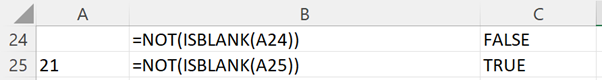In the table above, Cell A24 is empty, the formula =NOT(ISBLANK(A24)) returns the results as FALSE.

Cell A25 contains the number 21, it is not empty, and the formula =NOT(ISBLANK(A25)) returns the results as TRUE.